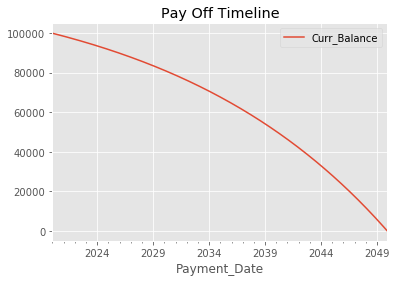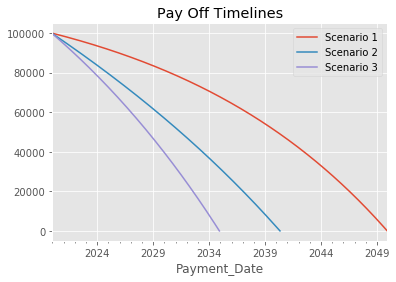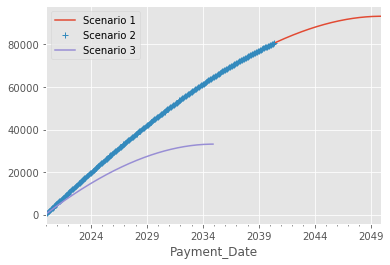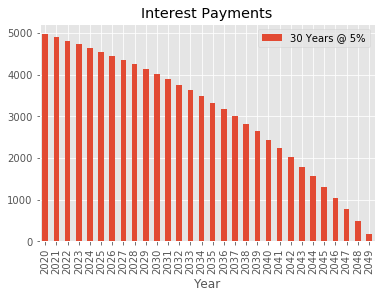# Amortization Example in Pandas¶

Code to support article at Practical Business Python

Setup the imports and matplotlib plotting

In :
import pandas as pd
import numpy as np
from datetime import date
import matplotlib.pyplot as plt
import matplotlib

In :
%matplotlib inline

In :
matplotlib.style.use('ggplot')


Define a function to build an amortization table/schedule and return the value as a pandas DataFrame

In :
def amortization_table(interest_rate, years, payments_year, principal, addl_principal=0, start_date=date.today()):
""" Calculate the amortization schedule given the loan details

Args:
interest_rate: The annual interest rate for this loan
years: Number of years for the loan
payments_year: Number of payments in a year
principal: Amount borrowed
must be a value less then 0, the function will convert a positive value to
negative
start_date (optional): Start date. Will start on first of next month if none provided

Returns:
schedule: Amortization schedule as a pandas dataframe
summary: Pandas dataframe that summarizes the payoff information
"""
# Ensure the additional payments are negative

# Create an index of the payment dates
rng = pd.date_range(start_date, periods=years * payments_year, freq='MS')
rng.name = "Payment_Date"

# Build up the Amortization schedule as a DataFrame
df = pd.DataFrame(index=rng,columns=['Payment', 'Principal', 'Interest',

# Add index by period (start at 1 not 0)
df.reset_index(inplace=True)
df.index += 1
df.index.name = "Period"

# Calculate the payment, principal and interests amounts using built in Numpy functions
per_payment = np.pmt(interest_rate/payments_year, years*payments_year, principal)
df["Payment"] = per_payment
df["Principal"] = np.ppmt(interest_rate/payments_year, df.index, years*payments_year, principal)
df["Interest"] = np.ipmt(interest_rate/payments_year, df.index, years*payments_year, principal)

# Round the values
df = df.round(2)

# Store the Cumulative Principal Payments and ensure it never gets larger than the original principal
df["Cumulative_Principal"] = df["Cumulative_Principal"].clip(lower=-principal)

# Calculate the current balance for each period
df["Curr_Balance"] = principal + df["Cumulative_Principal"]

# Determine the last payment date
try:
last_payment = df.query("Curr_Balance <= 0")["Curr_Balance"].idxmax(axis=1, skipna=True)
except ValueError:
last_payment = df.last_valid_index()

last_payment_date = "{:%m-%d-%Y}".format(df.loc[last_payment, "Payment_Date"])

# Truncate the data frame if we have additional principal payments:

# Remove the extra payment periods
df = df.loc[0:last_payment].copy()

# Calculate the principal for the last row
df.loc[last_payment, "Principal"] = -(df.loc[last_payment-1, "Curr_Balance"])

# Calculate the total payment for the last row
df.loc[last_payment, "Payment"] = df.loc[last_payment, ["Principal", "Interest"]].sum()

# Zero out the additional principal

# Get the payment info into a DataFrame in column order
payment_info = (df[["Payment", "Principal", "Addl_Principal", "Interest"]]
.sum().to_frame().T)

# Format the Date DataFrame
payment_details = pd.DataFrame.from_dict(dict([('payoff_date', [last_payment_date]),
('Interest Rate', [interest_rate]),
('Number of years', [years])
]))
# Add a column showing how much we pay each period.
# Combine addl principal with principal for total payment
payment_details["Period_Payment"] = round(per_payment, 2) + addl_principal

payment_summary = pd.concat([payment_details, payment_info], axis=1)
return df, payment_summary



## Examples of running the function¶

In :
schedule1, stats1 = amortization_table(0.05, 30, 12, 100000, addl_principal=0)


Take a look at the start and end of the table as well as the summary stats

In :
schedule1.head()

Out:
Payment_Date Payment Principal Interest Addl_Principal Curr_Balance Cumulative_Principal
Period
1 2020-01-01 -536.82 -120.15 -416.67 0 99879.85 -120.15
2 2020-02-01 -536.82 -120.66 -416.17 0 99759.19 -240.81
3 2020-03-01 -536.82 -121.16 -415.66 0 99638.03 -361.97
4 2020-04-01 -536.82 -121.66 -415.16 0 99516.37 -483.63
5 2020-05-01 -536.82 -122.17 -414.65 0 99394.20 -605.80
In :
schedule1.tail()

Out:
Payment_Date Payment Principal Interest Addl_Principal Curr_Balance Cumulative_Principal
Period
356 2049-08-01 -536.82 -525.78 -11.05 0 2125.09 -97874.91
357 2049-09-01 -536.82 -527.97 -8.85 0 1597.12 -98402.88
358 2049-10-01 -536.82 -530.17 -6.65 0 1066.95 -98933.05
359 2049-11-01 -536.82 -532.38 -4.45 0 534.57 -99465.43
360 2049-12-01 -536.82 -534.59 -2.23 0 0.00 -100000.00
In :
stats1

Out:
payoff_date Interest Rate Number of years Period_Payment Payment Principal Addl_Principal Interest
0 12-01-2049 0.05 30 -536.82 -193255.2 -100000.02 0.0 -93255.69

Try running some other scenarios and combining them into a single DataFrame

In :
schedule2, stats2 = amortization_table(0.05, 30, 12, 100000, addl_principal=-200)
schedule3, stats3 = amortization_table(0.04, 15, 12, 100000, addl_principal=0)

In :
# Combine all the scenarios into 1 view
pd.concat([stats1, stats2, stats3], ignore_index=True)

Out:
payoff_date Interest Rate Number of years Period_Payment Payment Principal Addl_Principal Interest
0 12-01-2049 0.05 30 -536.82 -193255.20 -100000.02 0.0 -93255.69
1 05-01-2040 0.05 30 -736.82 -131689.78 -51200.00 -48800.0 -80490.16
2 12-01-2034 0.04 15 -739.69 -133144.20 -100000.03 0.0 -33143.85
In :
schedule3.head()

Out:
Payment_Date Payment Principal Interest Addl_Principal Curr_Balance Cumulative_Principal
Period
1 2020-01-01 -739.69 -406.35 -333.33 0 99593.65 -406.35
2 2020-02-01 -739.69 -407.71 -331.98 0 99185.94 -814.06
3 2020-03-01 -739.69 -409.07 -330.62 0 98776.87 -1223.13
4 2020-04-01 -739.69 -410.43 -329.26 0 98366.44 -1633.56
5 2020-05-01 -739.69 -411.80 -327.89 0 97954.64 -2045.36

## Examples of plotting the data¶

In :
schedule1.plot(x='Payment_Date', y='Curr_Balance', title="Pay Off Timeline");In :
fig, ax = plt.subplots(1, 1)
schedule1.plot(x='Payment_Date', y='Curr_Balance', label="Scenario 1", ax=ax)
schedule2.plot(x='Payment_Date', y='Curr_Balance', label="Scenario 2", ax=ax)
schedule3.plot(x='Payment_Date', y='Curr_Balance', label="Scenario 3", ax=ax)
plt.title("Pay Off Timelines");In :
schedule1["Cum_Interest"] = schedule1["Interest"].abs().cumsum()
schedule2["Cum_Interest"] = schedule2["Interest"].abs().cumsum()
schedule3["Cum_Interest"] = schedule3["Interest"].abs().cumsum()

fig, ax = plt.subplots(1, 1)

schedule1.plot(x='Payment_Date', y='Cum_Interest', label="Scenario 1", ax=ax)
schedule2.plot(x='Payment_Date', y='Cum_Interest', label="Scenario 2", ax=ax, style='+')
schedule3.plot(x='Payment_Date', y='Cum_Interest', label="Scenario 3", ax=ax)

ax.legend(loc="best");In :
fig, ax = plt.subplots(1, 1)

y1_schedule = schedule1.set_index('Payment_Date').resample("A")["Interest"].sum().abs().reset_index()
y1_schedule["Year"] = y1_schedule["Payment_Date"].dt.year
y1_schedule.plot(kind="bar", x="Year", y="Interest", ax=ax, label="30 Years @ 5%")

plt.title("Interest Payments");In [ ]: